# 3 Data Visualization via ggplot2

We begin the development of your data science toolbox with data visualization. By visualizing our data, we will be able to gain valuable insights from our data that we couldn’t initially see from just looking at the raw data in spreadsheet form. We will use the ggplot2 package as it provides an easy way to customize your plots and is rooted in the data visualization theory known as The Grammar of Graphics (Wilkinson 2005).

At the most basic level, graphics/plots/charts (we use these terms interchangeably in this book) provide a nice way for us to get a sense for how quantitative variables compare in terms of their center (where the values tend to be located) and their spread (how they vary around the center). The most important thing to know about graphics is that they should be created to make it obvious for your audience to understand the findings and insight you want to get across. This does however require a balancing act. On the one hand, you want to highlight as many meaningful relationships and interesting findings as possible, but on the other you don’t want to include so many as to overwhelm your audience.

As we will see, plots/graphics also help us to identify patterns and outliers in our data. We will see that a common extension of these ideas is to compare the distribution of one quantitative variable (i.e., what the spread of a variable looks like or how the variable is distributed in terms of its values) as we go across the levels of a different categorical variable.

### Needed packages

Let’s load all the packages needed for this chapter (this assumes you’ve already installed them). Read Section 2.3 for information on how to install and load R packages.

library(nycflights13)
library(ggplot2)
library(dplyr)
library(knitr)

## 3.1 The Grammar of Graphics

We begin with a discussion of a theoretical framework for data visualization known as the “The Grammar of Graphics,” which serves as the basis for the ggplot2 package. Much like how we construct sentences in any language by using a linguistic grammar (nouns, verbs, subjects, objects, etc.), the theoretical framework given by Leland Wilkinson (Wilkinson 2005) allows us to specify the components of a statistical graphic.

### 3.1.1 Components of the Grammar

In short, the grammar tells us that:

A statistical graphic is a mapping of data variables to aesthetic attributes of geometric objects.

Specifically, we can break a graphic into the following three essential components:

1. data: the data-set comprised of variables that we map.
2. geom: the geometric object in question. This refers to our type of objects we can observe in our plot. For example, points, lines, bars, etc.
3. aes: aesthetic attributes of the geometric object that we can perceive on a graphic. For example, x/y position, color, shape, and size. Each assigned aesthetic attribute can be mapped to a variable in our data-set.

Let’s break down the grammar with an example.

### 3.1.2 Gapminder

In February 2006, a statistician named Hans Rosling gave a TED talk titled “The best stats you’ve ever seen” where he presented global economic, health, and development data from the website gapminder.org. For example, from the 1704 countries included from 2007, consider only the first 6 countries when listed alphabetically:

Table 3.1: Gapminder 2007 Data
Country Continent Life Expectancy Population GDP per Capita
Afghanistan Asia 43.83 31889923 974.6
Albania Europe 76.42 3600523 5937.0
Algeria Africa 72.30 33333216 6223.4
Angola Africa 42.73 12420476 4797.2
Argentina Americas 75.32 40301927 12779.4
Australia Oceania 81.23 20434176 34435.4

Each row in this table corresponds to a country in 2007. For each row, we have 5 columns:

1. Country: Name of country.
2. Continent: Which of the five continents the country is part of. (Note that Americas groups North and South America and that Antarctica is excluded here.)
3. Life Expectancy: Life expectancy in years.
4. Population: Number of people living in the country.
5. GDP per Capita: Gross domestic product (in US dollars).

Now consider Figure 3.1, which plots this data for all 142 countries in the data frame. Note that R will deal with large numbers using scientific notation. So in the legend for “Population”, 1.25e+09 = $$1.25 \times 10^{9}$$ = 1,250,000,000 = 1.25 billion.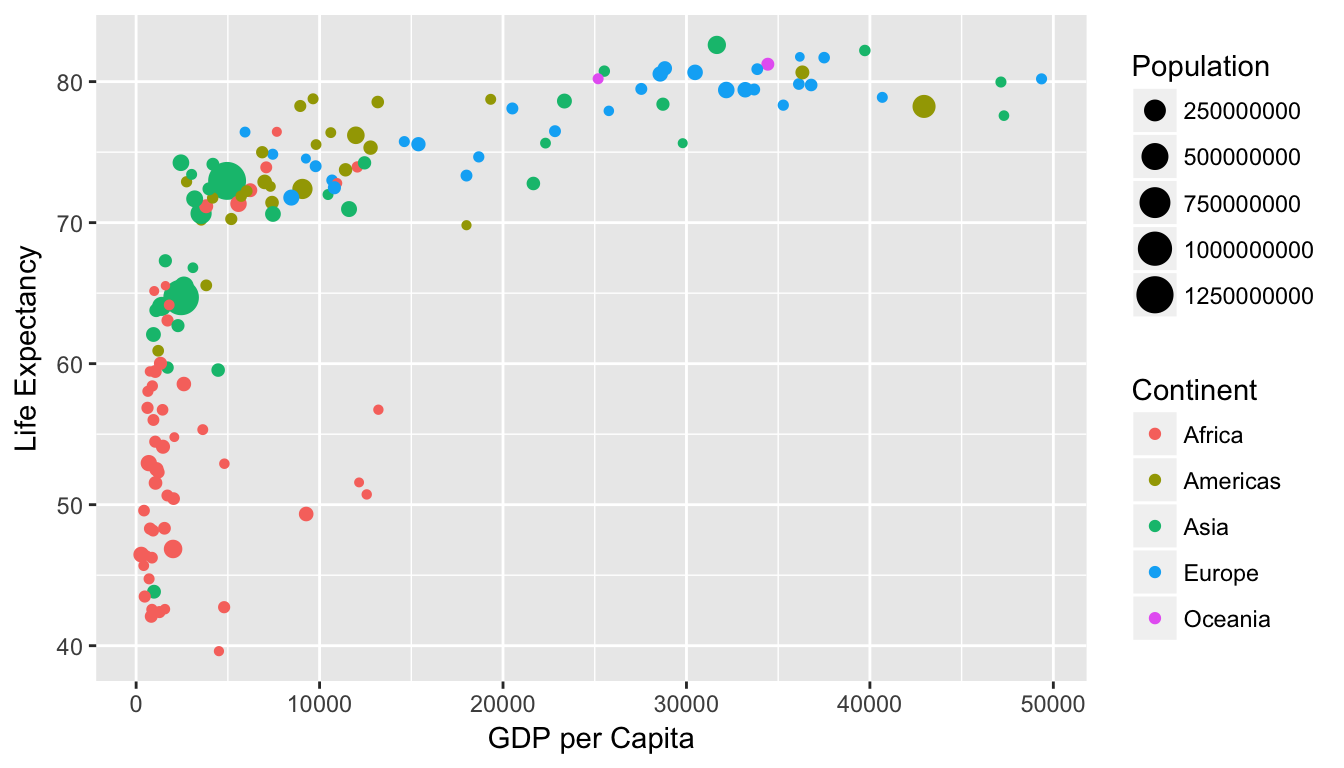Figure 3.1: Life Expectancy over GDP per Capita in 2007

Let’s view this plot through the grammar of graphics:

1. The data variable GDP per Capita gets mapped to the x-position aesthetic of the points.
2. The data variable Life Expectancy gets mapped to the y-position aesthetic of the points.
3. The data variable Population gets mapped to the size aesthetic of the points.
4. The data variable Continent gets mapped to the color aesthetic of the points.

Recall that data here corresponds to each of the variables being in the same data frame and the “data variable” corresponds to a column in a data frame.

While in this example we are considering one type of geometric object (of type point), graphics are not limited to just points. Some plots involve lines while others involve bars. Let’s summarize the three essential components of the grammar in a table:

Table 3.2: Summary of Grammar of Graphics for this plot
data variable aes geom
GDP per Capita x point
Life Expectancy y point
Population size point
Continent color point

### 3.1.3 Other components of the Grammar

There are other components of the Grammar of Graphics we can control. As you start to delve deeper into the Grammar of Graphics, you’ll start to encounter these topics more and more often. In this book, we’ll only work with the two other components below (The other components are left to a more advanced text such as R for Data Science (Grolemund and Wickham 2016)):

• facetting breaks up a plot into small multiples corresponding to the levels of another variable (Section 3.6)
• position adjustments for barplots (Section 3.8)

In general, the Grammar of Graphics allows for a high degree of customization and also a consistent framework for easy updating/modification of plots.

### 3.1.4 The ggplot2 package

In this book, we will be using the ggplot2 package for data visualization, which is an implementation of the Grammar of Graphics for R (Wickham and Chang 2016). You may have noticed that a lot of the previous text in this chapter is written in computer font. This is because the various components of the Grammar of Graphics are specified in the ggplot function, which expects at a bare minimal as arguments:

• The data frame where the variables exist: the data argument
• The mapping of the variables to aesthetic attributes: the mapping argument, which specifies the aesthetic attributes involved

After we’ve specified these components, we then add layers to the plot. The most essential layer to add to a plot is the specification of which type of geometric object we want the plot to involve; e.g. points, lines, bars. Other layers we can add include the specification of the plot title, axes labels, and visual themes for the plot.

Let’s now put the theory of the Grammar of Graphics into practice.

## 3.2 Five Named Graphs - The 5NG

For our purposes, we will be limiting consideration to five different types of graphs. We term these five named graphs the 5NG:

1. scatterplots
2. linegraphs
3. boxplots
4. histograms
5. barplots

We will discuss some variations of these plots, but with this basic repertoire in your toolbox you can visualize a wide array of different data variable types. Note that certain plots are only appropriate for categorical/logical variables and others only for quantitative variables. You’ll want to quiz yourself often as we go along on which plot makes sense a given a particular problem or data-set.

## 3.3 5NG#1: Scatterplots

The simplest of the 5NG are scatterplots (also called bivariate plots); they allow you to investigate the relationship between two continuous variables. While you may already be familiar with this type of plot, let’s view it through the lens of the Grammar of Graphics. Specifically, we will graphically investigate the relationship between the following two continuous variables in the flights data frame:

1. dep_delay: departure delay on the horizontal “x” axis and
2. arr_delay: arrival delay on the vertical “y” axis

for Alaska Airlines flights leaving NYC in 2013. This requires paring down the flights data frame to a smaller data frame all_alaska_flights consisting of only Alaska Airlines (carrier code “AS”) flights.

all_alaska_flights <- flights %>%
filter(carrier == "AS")

This code snippet makes use of functions in the dplyr package for data wrangling to achieve our goal: it takes the flights data frame and filters it to only return the rows which meet the condition carrier == "AS". Recall from Section 2.2 that testing for equality is specified with == and not =. You will see many more examples of == and filter() in Chapter 5.

Learning check

(LC3.1) Take a look at both the flights and all_alaska_flights data frames by running View(flights) and View(all_alaska_flights) in the console. In what respect do these data frames differ?

### 3.3.1 Scatterplots via geom_point

We proceed to create the scatterplot using the ggplot() function:

ggplot(data = all_alaska_flights, aes(x = dep_delay, y = arr_delay)) +
geom_point()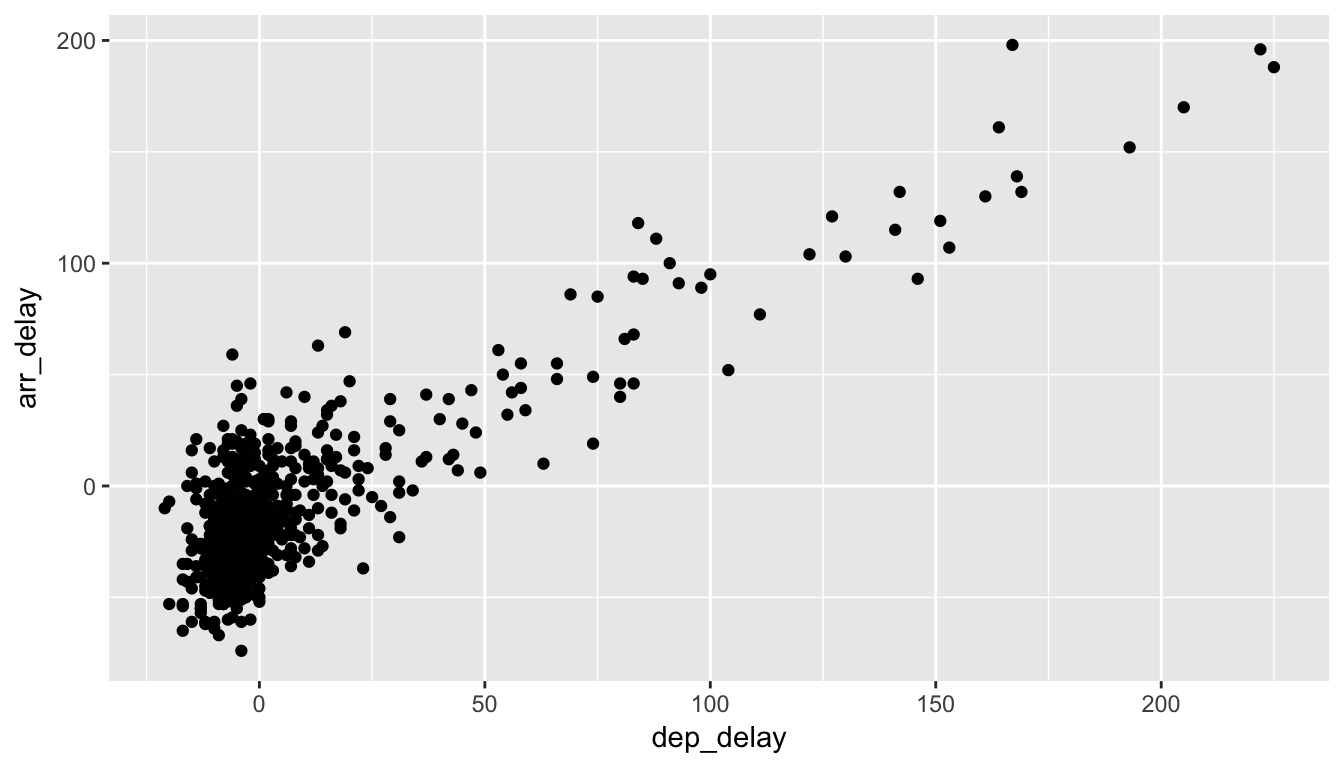Figure 3.2: Arrival Delays vs Departure Delays for Alaska Airlines flights from NYC in 2013

In Figure 3.2 we see that a positive relationship exists between dep_delay and arr_delay: as departure delays increase, arrival delays tend to also increase. We also note that the majority of points fall near the point (0, 0). There is a large mass of points clustered there. Let’s break this down, keeping in mind our discussion in Section 3.1:

• Within the ggplot() function call, we specify two of the components of the grammar:
1. The data frame to be all_alaska_flights by setting data = all_alaska_flights
2. The aesthetic mapping by setting aes(x = dep_delay, y = arr_delay). Specifically
• the variable dep_delay maps to the x position aesthetic
• the variable arr_delay maps to the y position aesthetic
• We add a layer to the ggplot() function call using the + sign. The layer in question specifies the third component of the grammar: the geometric object. In this case the geometric object are points, set by specifying geom_point().

Some notes on layers:

• Note that the + sign comes at the end of lines, and not at the beginning. You’ll get an error in R if you put it at the beginning.
• When adding layers to a plot, you are encouraged to hit Return on your keyboard after entering the + so that the code for each layer is on a new line. As we add more and more layers to plots, you’ll see this will greatly improve the legibility of your code.
• To stress the importance of adding layers, in particular the layer specifying the geometric object, consider Figure 3.3 where no layers are added. A not very useful plot!
ggplot(data = all_alaska_flights, mapping = aes(x = dep_delay, y = arr_delay))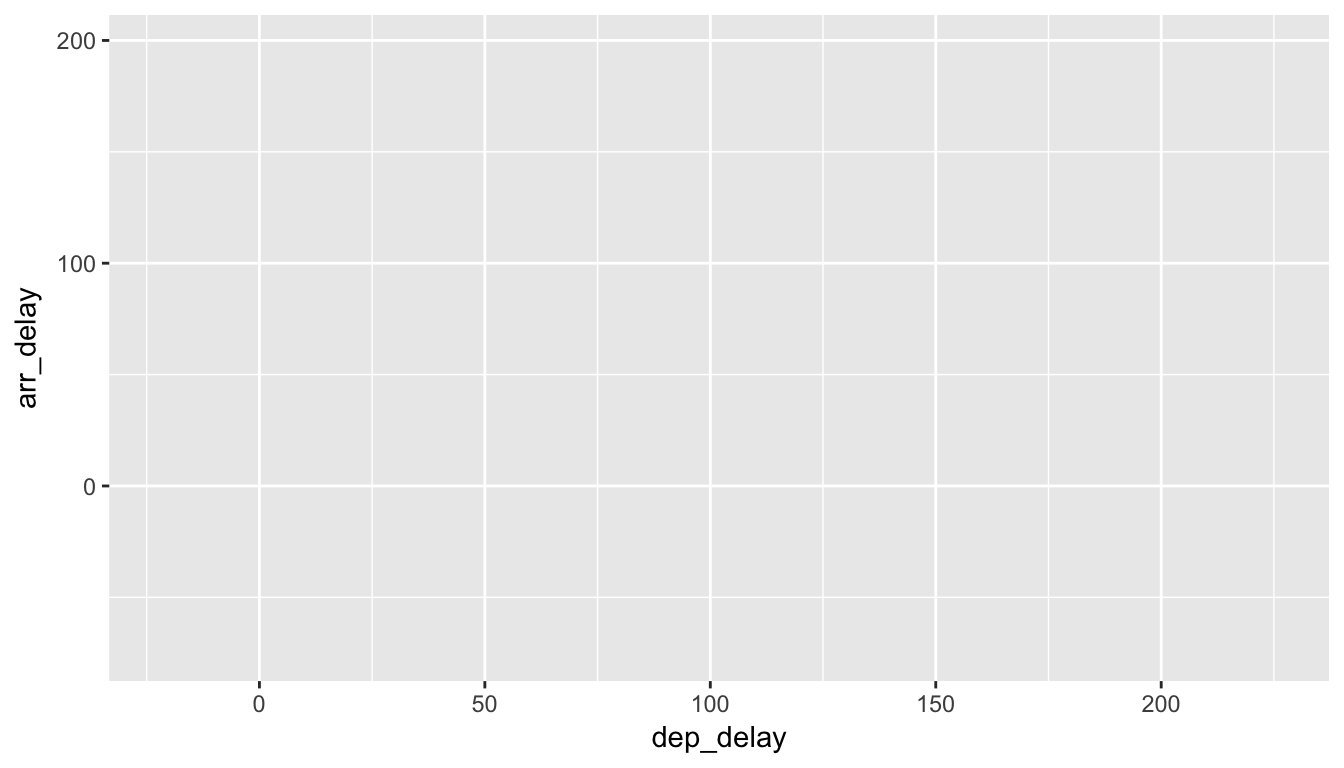Figure 3.3: Plot with No Layers

Learning check

(LC3.2) What are some practical reasons why dep_delay and arr_delay have a positive relationship?

(LC3.3) What variables (not necessarily in the flights data frame) would you expect to have a negative correlation (i.e. a negative relationship) with dep_delay? Why? Remember that we are focusing on continuous variables here.

(LC3.4) Why do you believe there is a cluster of points near (0, 0)? What does (0, 0) correspond to in terms of the Alaskan flights?

(LC3.5) What are some other features of the plot that stand out to you?

(LC3.6) Create a new scatterplot using different variables in the all_alaska_flights data frame by modifying the example above.

### 3.3.2 Over-plotting

The large mass of points near (0, 0) in Figure 3.2 can cause some confusion. This is the result of a phenomenon called over-plotting. As one may guess, this corresponds to values being plotted on top of each other over and over again. It is often difficult to know just how many values are plotted in this way when looking at a basic scatterplot as we have here. There are two ways to address this issue:

1. By adjusting the transparency of the points via the alpha argument
2. By jittering the points via geom_jitter()

The first way of relieving over-plotting is by changing the alpha argument in geom_point() which controls the transparency of the points. By default, this value is set to 1. We can change this to any value between 0 and 1 where 0 sets the points to be 100% transparent and 1 sets the points to be 100% opaque. Note how the following function call is identical to the one in Section 3.3, but with alpha = 0.2 added to the geom_point().

ggplot(data = all_alaska_flights, mapping = aes(x = dep_delay, y = arr_delay)) +
geom_point(alpha = 0.2)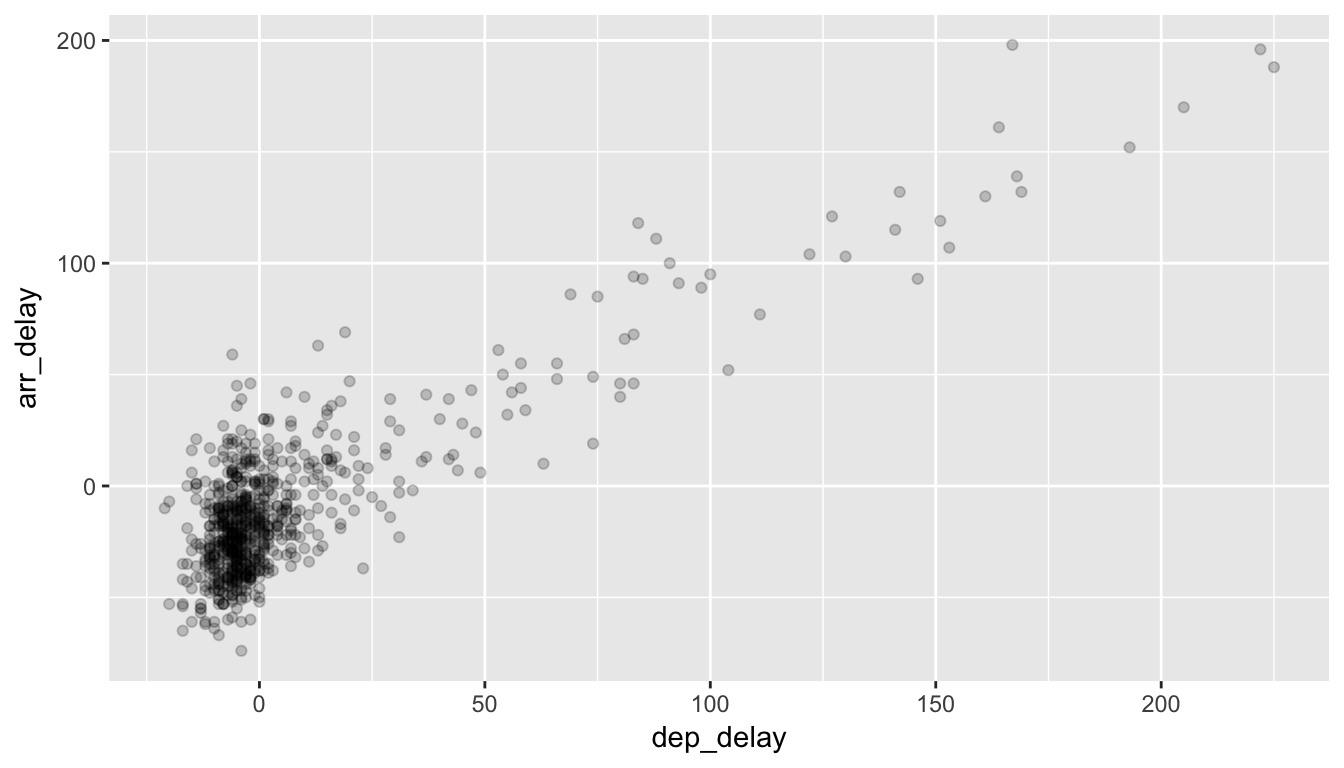Figure 3.4: Delay scatterplot with alpha=0.2

The key feature to note in Figure 3.4 is that the transparency of the points is cumulative: areas with a high-degree of over-plotting are darker, whereas areas with a lower degree are less dark.

Note that there is no aes() surrounding alpha = 0.2 here. Since we are NOT mapping a variable to an aesthetic but instead are just changing a setting, we don’t need to create a mapping with aes(). In fact, you’ll receive an error if you try to change the second line above to geom_point(aes(alpha = 0.2)).

The second way of relieving over-plotting is to jitter the points a bit. In other words, we are going to add just a bit of random noise to the points to better see them and remove some of the over-plotting. You can think of “jittering” as shaking the points around a bit on the plot. Instead of using geom_point, we use geom_jitter to perform this shaking. To specify how much jitter to add, we adjust the width and height arguments. This corresponds to how hard you’d like to shake the plot in units corresponding to those for both the horizontal and vertical variables (in this case, minutes).

ggplot(all_alaska_flights, aes(x = dep_delay, y = arr_delay)) +
geom_jitter(width = 30, height = 30)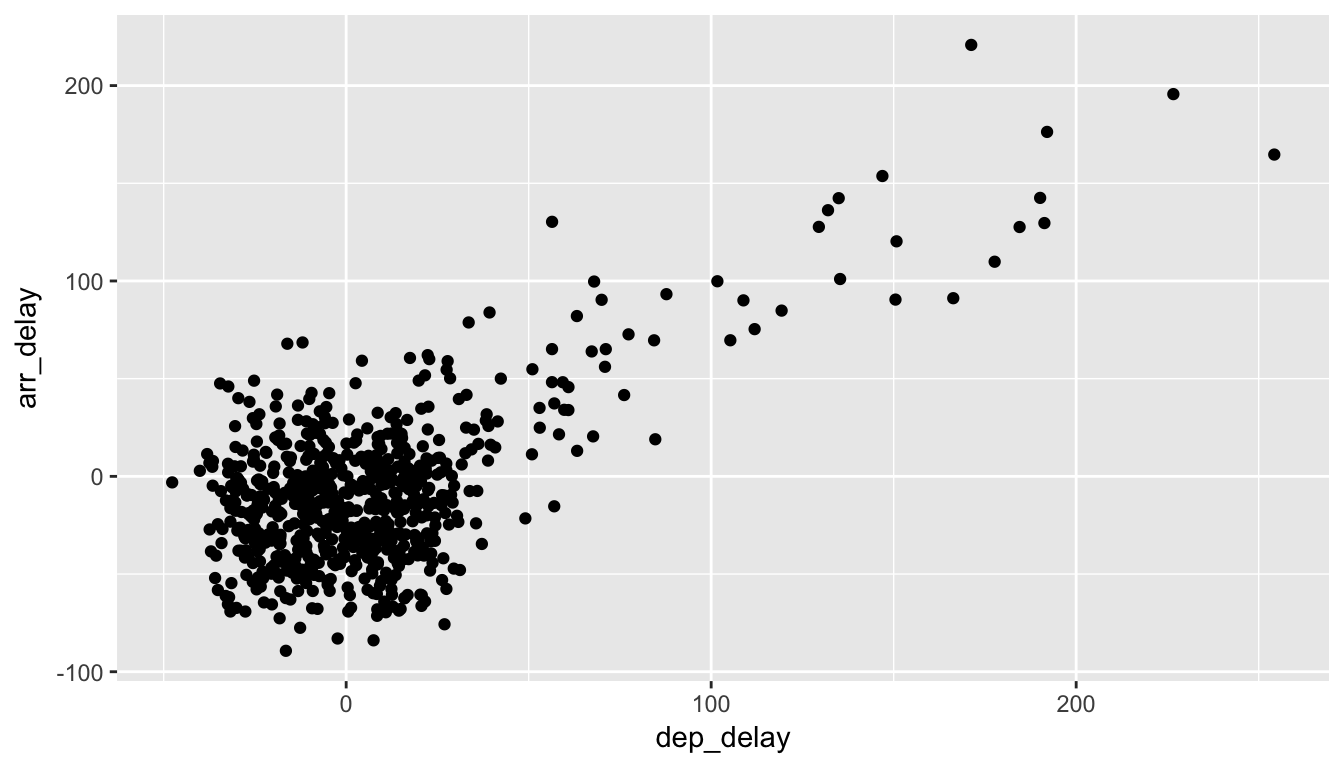Figure 3.5: Jittered delay scatterplot

Note how this function call is identical to the one in Subsection 3.3.1, but with geom_point() replaced with geom_jitter(). The plot in Figure 3.5 helps us a little bit in getting a sense for the over-plotting, but with a relatively large data-set like this one (714 flights), it can be argued that changing the transparency of the points by setting alpha proved more effective.

You may have noticed that in the code to create Figure 3.5 have also dropped the data = and also the mapping = code before aes in this example. Since ggplot is expecting its first argument data to be a data frame and its second argument to correspond to mapping =, you can omit both and you’ll get the same plot. As you get more and more practice, you’ll likely find yourself not including the specification of the argument like this. It’s good practice to always include it though, especially as you are just beginning to practice with R code.

Learning check

(LC3.7) Why is setting the alpha argument value useful with scatterplots? What further information does it give you that a regular scatterplot cannot?

(LC3.8) After viewing the Figure 3.4 above, give an approximate range of arrival times and departure times that occur the most frequently. How has that region changed compared to when you observed the same plot without the alpha = 0.2 set in Figure 3.2?

### 3.3.3 Summary

Scatterplots display the relationship between two continuous variables. They are among the most commonly used plots because they can provide an immediate way to see the trend in one variable versus another. However, if you try to create a scatterplot where either one of the two variables is not quantitative, you will get strange results. Be careful!

With medium to large data-sets, you may need to play with either geom_jitter() or the alpha argument in order to get a good feel for relationships in your data. This tweaking is often a fun part of data visualization since you’ll have the chance to see different relationships come about as you make subtle changes to your plots.

## 3.4 5NG#2: Linegraphs

The next of the 5NG is a linegraph. They are most frequently used when the x-axis represents time and the y-axis represents some other numerical variable; such plots are known as time series. Time represents a variable that is connected together by each day following the previous day. In other words, time has a natural ordering. Linegraphs should be avoided when there is not a clear sequential ordering to the explanatory variable, i.e. the x-variable or the predictor variable.

Our focus now turns to the temp variable in this weather data-set. By

• Looking over the weather data-set by typing View(weather) in the console.
• Running ?weather to bring up the help file.

We can see that the temp variable corresponds to hourly temperature (in Fahrenheit) recordings at weather stations near airports in New York City. Instead of considering all hours in 2013 for all three airports in NYC, let’s focus on the hourly temperature at Newark airport (origin code “EWR”) for the first 15 days in January 2013. The weather data frame in the nycflights13 package contains this data, but we first need to filter it to only include those rows that correspond to Newark in the first 15 days of January.

early_january_weather <- weather %>%
filter(origin == "EWR" & month == 1 & day <= 15)

This is similar to the previous use of the filter command in Section 3.3, however we now use the & operator. The above selects only those rows in weather where the originating airport is "EWR" and we are in the first month and the day is from 1 to 15 inclusive.

Learning check

(LC3.9) Take a look at both the weather and early_january_weather data frames by running View(weather) and View(early_january_weather) in the console. In what respect do these data frames differ?

(LC3.10) The weather data is recorded hourly. Why does the time_hour variable correctly identify the hour of the measurement whereas the hour variable does not?

### 3.4.1 Linegraphs via geom_line

We plot a linegraph of hourly temperature using geom_line():

ggplot(data = early_january_weather, aes(x = time_hour, y = temp)) +
geom_line()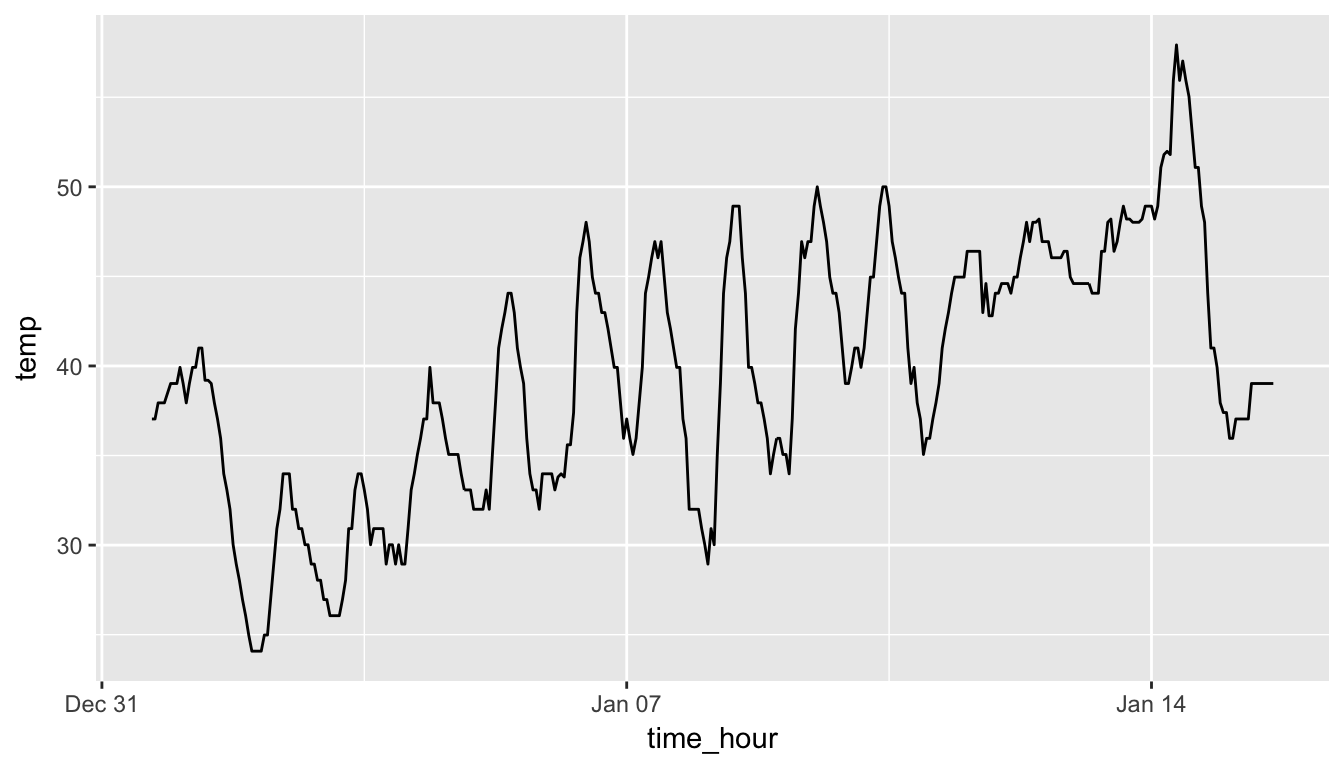Figure 3.6: Hourly Temperature in Newark for January 1-15, 2013

Much as with the ggplot() call in Chapter 3.3.1, we describe the components of the Grammar of Graphics:

• Within the ggplot() function call, we specify two of the components of the grammar:
1. The data frame to be early_january_weather by setting data = early_january_weather
2. The aesthetic mapping by setting aes(x = time_hour, y = temp). Specifically
• time_hour (i.e. the time variable) maps to the x position
• temp maps to the y position
• We add a layer to the ggplot() function call using the + sign
• The layer in question specifies the third component of the grammar: the geometric object in question. In this case the geometric object is a line, set by specifying geom_line().

Learning check

(LC3.11) Why should linegraphs be avoided when there is not a clear ordering of the horizontal axis?

(LC3.12) Why are linegraphs frequently used when time is the explanatory variable?

(LC3.13) Plot a time series of a variable other than temp for Newark Airport in the first 15 days of January 2013.

### 3.4.2 Summary

Linegraphs, just like scatterplots, display the relationship between two continuous variables. However, the variable on the x-axis (i.e. the explanatory variable) should have a natural ordering, like some notion of time. We can mislead our audience if that isn’t the case.

## 3.5 5NG#3: Histograms

Let’s consider the temp variable in the weather data frame once again, but now unlike with the linegraphs in Chapter 3.4, let’s say we don’t care about the relationship of temperature to time, but rather we care about the (statistical) distribution of temperatures. We could just produce points where each of the different values appear on something similar to a number line: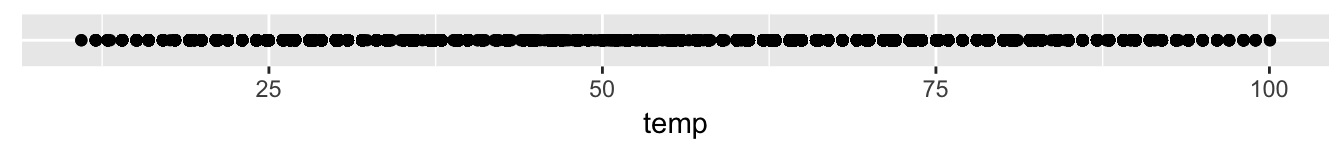Figure 3.7: Plot of Hourly Temperature Recordings from NYC in 2013

This gives us a general idea of how the values of temp differ. We see that temperatures vary from around 11 up to 100 degrees Fahrenheit. The area between 40 and 60 degrees appears to have more points plotted than outside that range.

### 3.5.1 Histograms via geom_histogram

What is commonly produced instead of the above plot is a plot known as a histogram. The histogram shows how many elements of a single numerical variable fall in specified bins. In this case, these bins may correspond to between 0-10°F, 10-20°F, etc. We produce a histogram of the hour temperatures at all three NYC airports in 2013:

ggplot(data = weather, mapping = aes(x = temp)) +
geom_histogram()
stat_bin() using bins = 30. Pick better value with binwidth.
Warning: Removed 1 rows containing non-finite values (stat_bin).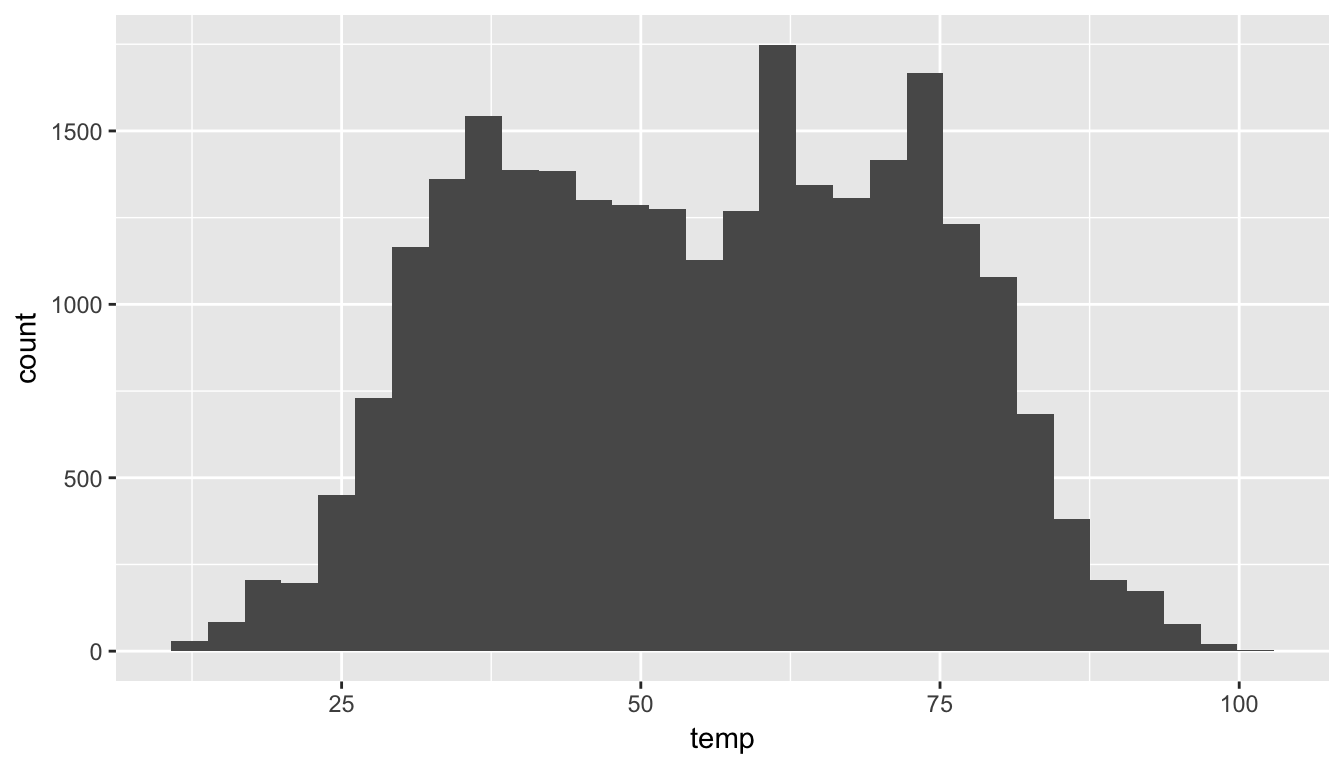Figure 3.8: Histogram of Hourly Temperature Recordings from NYC in 2013

Note here:

• There is only one variable being mapped in aes(): the single continuous variable temp. You don’t need to compute the y-aesthetic: it gets computed automatically.
• We set the geometric object to be geom_histogram()
• We got a warning message of 1 rows containing non-finite values being removed. This is due to one of the values of temperature being missing. R is alerting us that this happened.
• Another warning corresponds to an urge to specify the number of bins you’d like to create.

We can adjust characteristics of the bins in one of two ways:

1. By adjusting the number of bins via the bins argument
2. By adjusting the width of the bins via the binwidth argument

First, we have the power to specify how many bins we would like to put the data into as an argument in the geom_histogram() function. By default, this is chosen to be 30 somewhat arbitrarily; we have received a warning above our plot that this was done.

ggplot(data = weather, mapping = aes(x = temp)) +
geom_histogram(bins = 60, color = "white")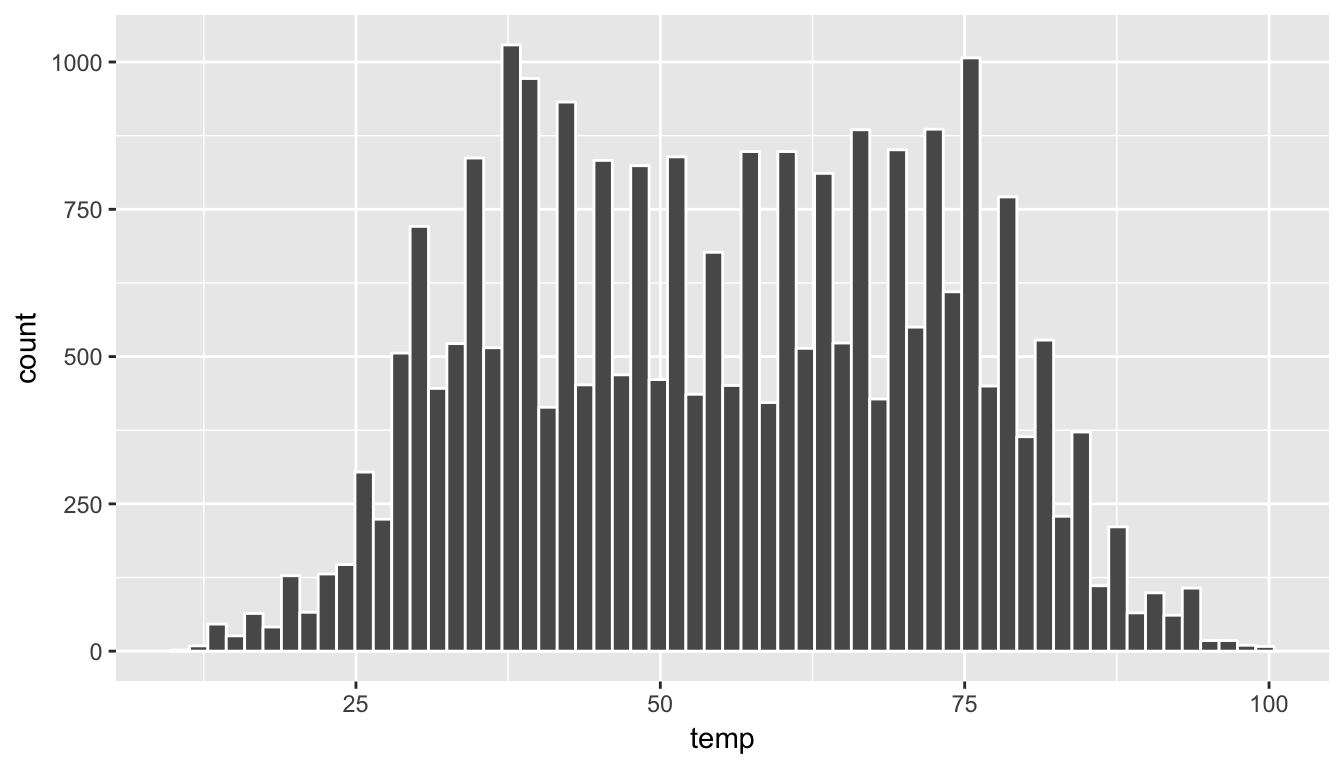Figure 3.9: Histogram of Hourly Temperature Recordings from NYC in 2013 - 60 Bins

Note the addition of the color argument. If you’d like to be able to more easily differentiate each of the bins, you can specify the color of the outline as done above.

Second, instead of specifying the number of bins, we can also specify the width of the bins by using the binwidth argument in the geom_histogram function.

ggplot(data = weather, mapping = aes(x = temp)) +
geom_histogram(binwidth = 10, color = "white")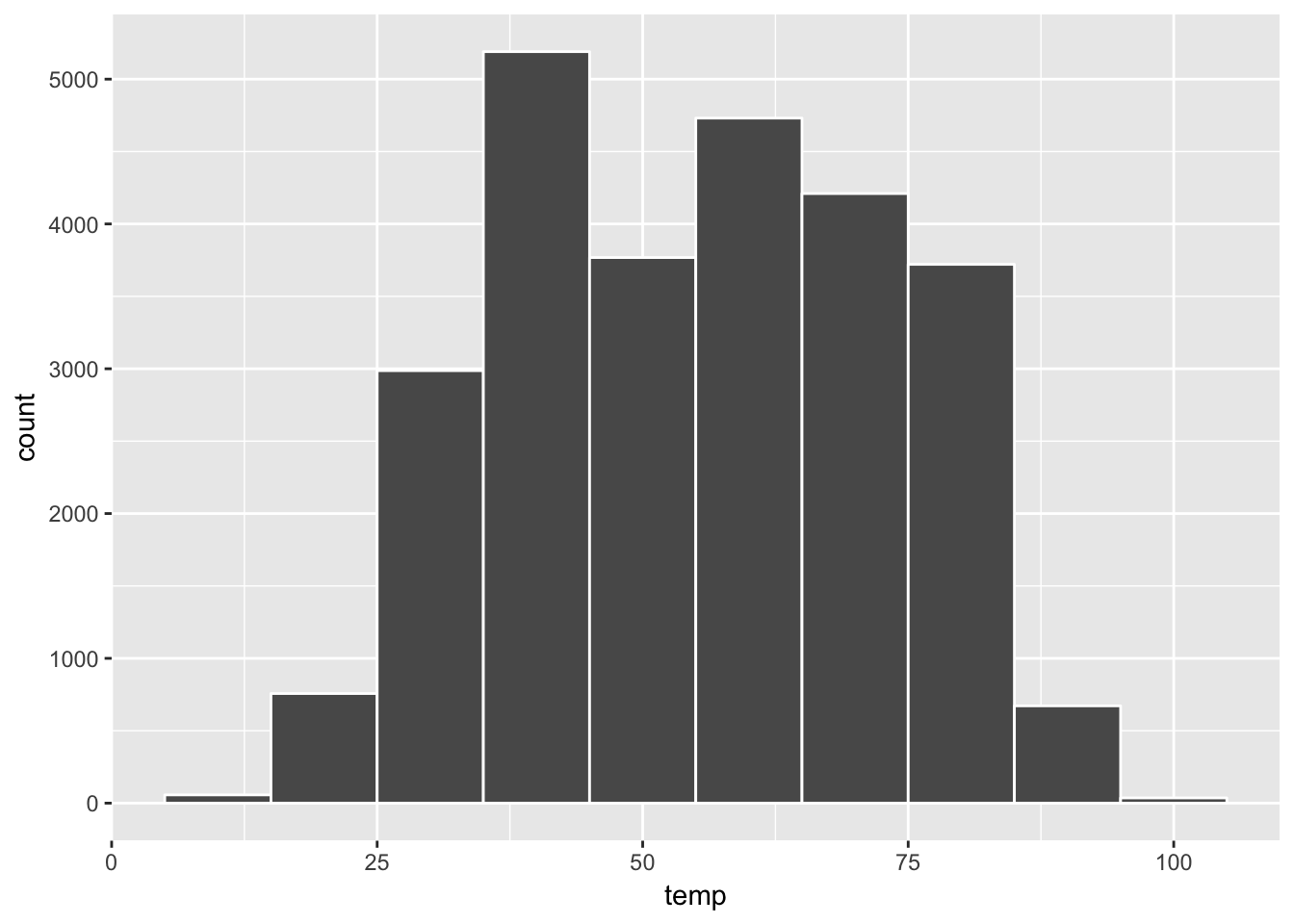Figure 3.10: Histogram of Hourly Temperature Recordings from NYC in 2013 - Binwidth = 10

Learning check

(LC3.14) What does changing the number of bins from 30 to 60 tell us about the distribution of temperatures?

(LC3.15) Would you classify the distribution of temperatures as symmetric or skewed?

(LC3.16) What would you guess is the “center” value in this distribution? Why did you make that choice?

(LC3.17) Is this data spread out greatly from the center or is it close? Why?

### 3.5.3 Summary

Histograms, unlike scatterplots and linegraphs, present information on only a single continuous variable. In particular they are visualizations of the (statistical) distribution of values.

## 3.6 Facets

Before continuing the 5NG, we briefly introduce a new concept called faceting. Faceting is used when we’d like to create small multiples of the same plot over a different categorical variable. By default, all of the small multiples will have the same vertical axis.

For example, suppose we were interested in looking at how the temperature histograms we saw in Chapter 3.5 varied by month. This is what is meant by “the distribution of a variable over another variable”: temp is one variable and month is the other variable. In order to look at histograms of temp for each month, we add a layer facet_wrap(~ month). You can also specify how many rows you’d like the small multiple plots to be in using nrow or how many columns using ncol inside of facet_wrap.

ggplot(data = weather, aes(x = temp)) +
geom_histogram(binwidth = 5, color = "white") +
facet_wrap(~ month, nrow = 4)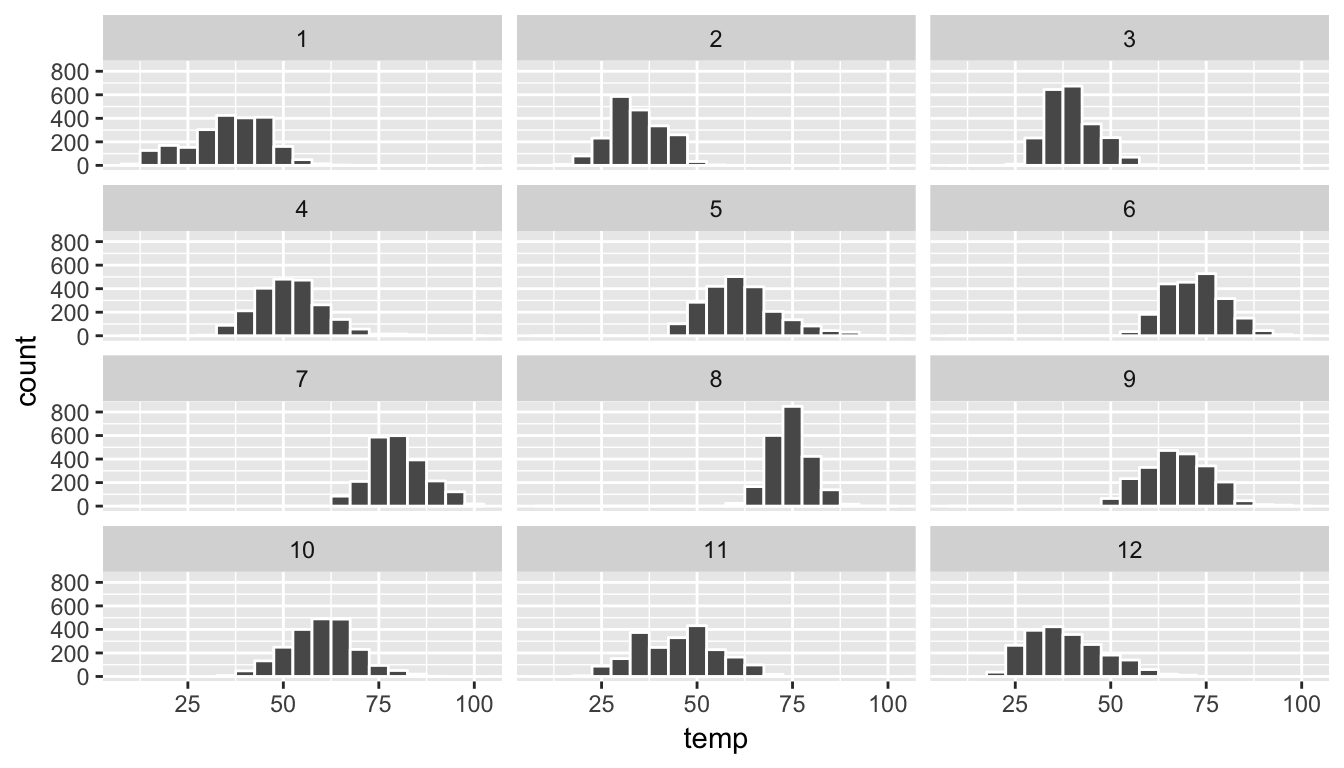Figure 3.11: Faceted histogram

Note the use of the ~ before month in facet_wrap. The tilde (~) is required and you’ll receive the error Error in as.quoted(facets) : object 'month' not found if you don’t include it before month here.

As we might expect, the temperature tends to increase as summer approaches and then decrease as winter approaches.

Learning check

(LC3.18) What other things do you notice about the faceted plot above? How does a faceted plot help us see relationships between two variables?

(LC3.19) What do the numbers 1-12 correspond to in the plot above? What about 25, 50, 75, 100?

(LC3.20) For which types of data-sets would these types of faceted plots not work well in comparing relationships between variables? Give an example describing the nature of these variables and other important characteristics.

(LC3.21) Does the temp variable in the weather data-set have a lot of variability? Why do you say that?

## 3.7 5NG#4: Boxplots

While using faceted histograms can provide a way to compare distributions of a continuous variable split by groups of a categorical variable as in Section 3.6, an alternative plot called a boxplot (also called a side-by-side boxplot) achieves the same task and is frequently preferred. The boxplot uses the information provided in the five-number summary referred to in Appendix A. It gives a way to compare this summary information across the different levels of a categorical variable.

### 3.7.1 Boxplots via geom_boxplot

Let’s create a boxplot to compare the monthly temperatures as we did above with the faceted histograms.

ggplot(data = weather, aes(x = month, y = temp)) +
geom_boxplot()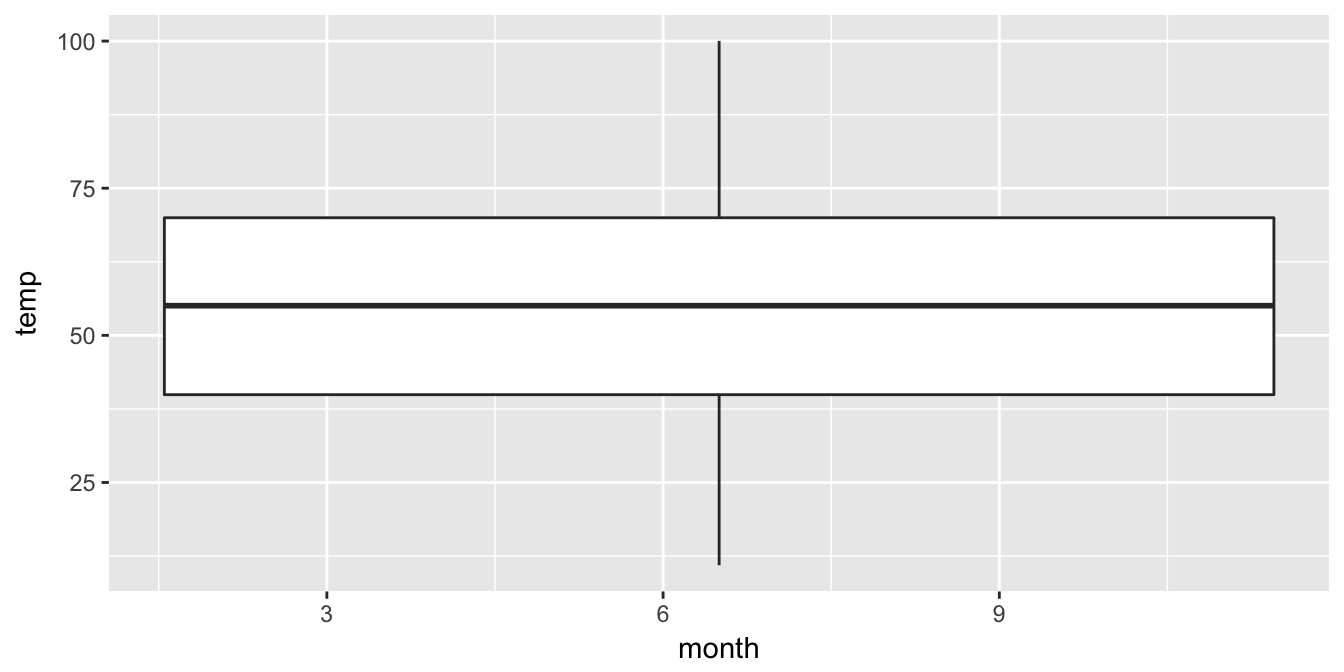Figure 3.12: Invalid boxplot specification

Warning messages:
1: Continuous x aesthetic -- did you forget aes(group=...)?
2: Removed 1 rows containing non-finite values (stat_boxplot). 

Note the set of warnings that is given here. The second warning corresponds to missing values in the data frame and it is turned off on subsequent plots. Let’s focus on the first warning.

Observe that this plot does not look like what we were expecting. We were expecting to see the distribution of temperatures for each month (so 12 different boxplots). The first warning is letting us know that we are plotting a continuous, and not categorical variable, on the x-axis. This gives us the overall boxplot without any other groupings. We can get around this by introducing a new function for our x variable:

ggplot(data = weather, mapping = aes(x = factor(month), y = temp)) +
geom_boxplot()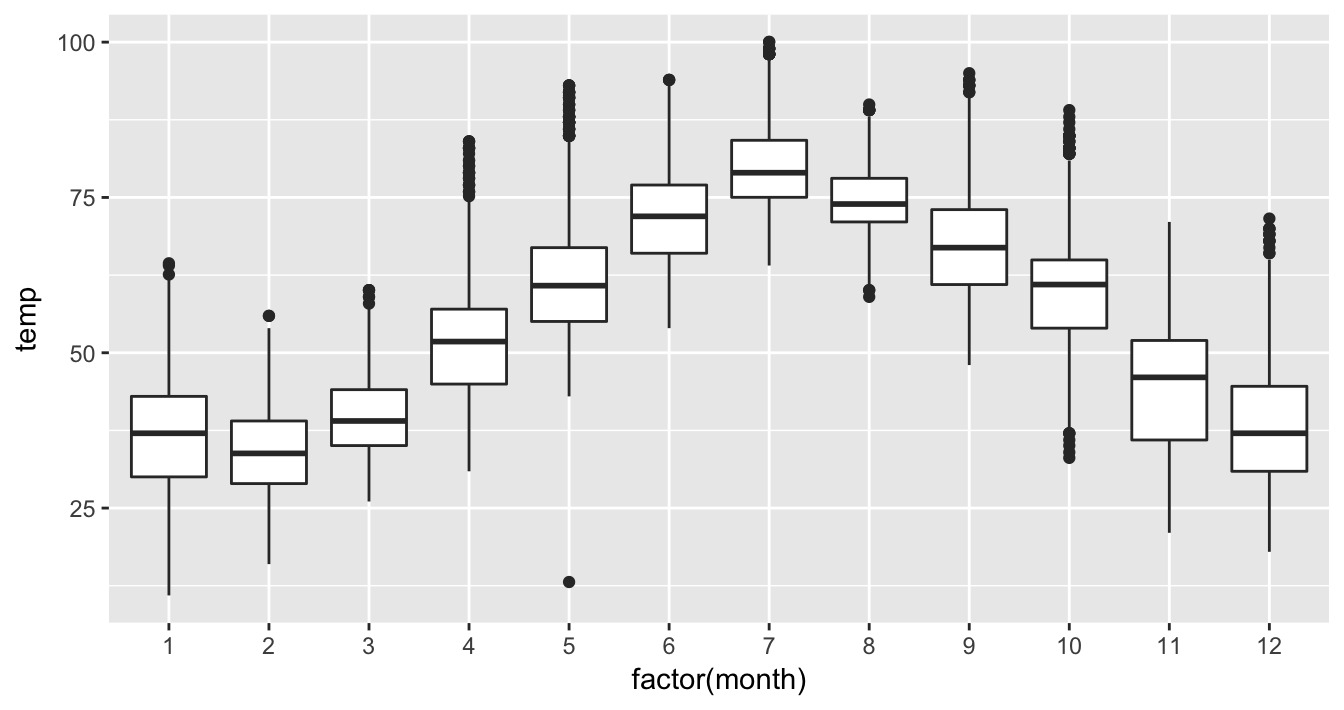Figure 3.13: Month by temp boxplot

We have introduced a new function called factor() here. One of the things this function does is to convert a discrete value like month (1, 2, …, 12) into a categorical variable. The “box” part of this plot represents the 25th percentile, the median (50th percentile), and the 75th percentile. The dots correspond to outliers. (The specific formulation for these outliers is discussed in Appendix A.) The lines show how the data varies that is not in the center 50% defined by the first and third quantiles. Longer lines correspond to more variability and shorter lines correspond to less variability.

Learning check

(LC3.22) What does the dot at the bottom of the plot for May correspond to? Explain what might have occurred in May to produce this point.

(LC3.23) Which months have the highest variability in temperature? What reasons do you think this is?

(LC3.24) We looked at the distribution of a continuous variable over a categorical variable here with this boxplot. Why can’t we look at the distribution of one continuous variable over the distribution of another continuous variable? Say, temperature across pressure, for example?

(LC3.25) Boxplots provide a simple way to identify outliers. Why may outliers be easier to identify when looking at a boxplot instead of a faceted histogram?

### 3.7.2 Summary

Boxplots provide a way to compare and contrast the distribution of one quantitative variable across multiple levels of one categorical variable. One can see where the median falls across the different groups by looking at the center line in the box. To see how spread out the variable is across the different groups, look at both the width of the box and also how far the lines stretch vertically from the box. (If the lines stretch far from the box but the box has a small width, the variability of the values closer to the center is much smaller than the variability of the outer ends of the variable.) Outliers are even more easily identified when looking at a boxplot than when looking at a histogram.

## 3.8 5NG#5: Barplots

Both histograms and boxplots represent ways to visualize the variability of continuous variables. Another common task is to present the distribution of a categorical variable. This is a simpler task, focused on how many elements from the data fall into different categories of the categorical variable. Often the best way to visualize these different counts (also known as frequencies) is via a barplot, also known as a barchart.

One complication, however, is how your counts are represented in your data. For example, run the following code in your Console. This code manually creates two data frames representing counts of fruit.

fruits <- data_frame(
fruit = c("apple", "apple", "apple", "orange", "orange")
)
fruits_counted <- data_frame(
fruit = c("apple", "orange"),
number = c(3, 2)
)

We see both the fruits and fruits_counted data frames represent the same collection of fruit: three apples and two bananas. However, whereas fruits just lists the fruit:

fruit
apple
apple
apple
orange
orange

fruits_counted has a variable count, where the counts are pre-tabulated.

Table 3.3: Fruits (Pre-Counted)
fruit number
apple 3
orange 2

Compare the barcharts in Figures 3.14 and 3.15, which are identical, but are based on two different data frames:

ggplot(data = fruits, mapping = aes(x = fruit)) +
geom_bar()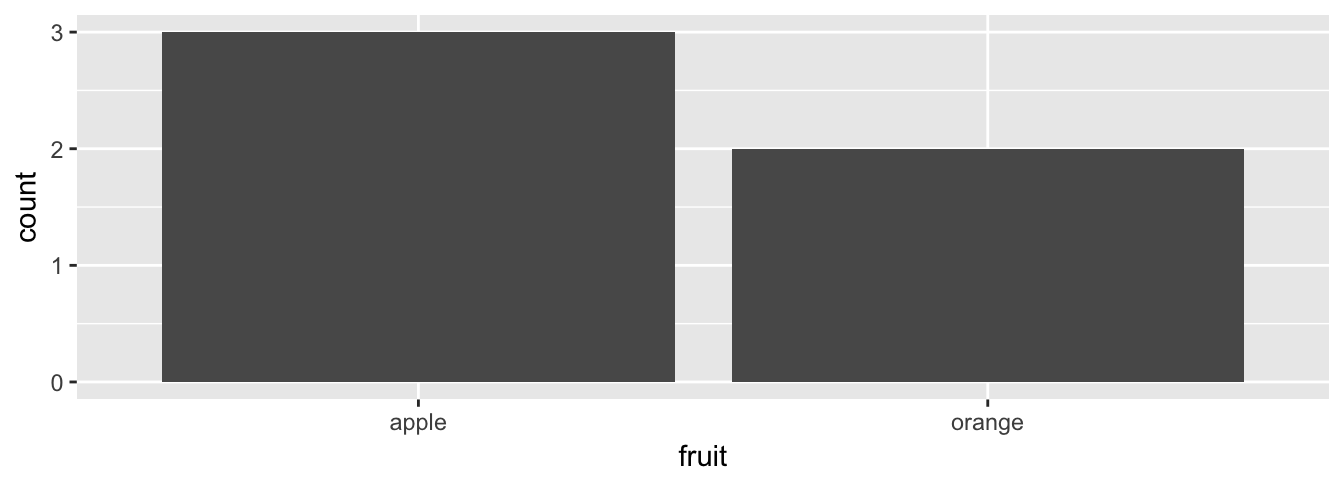Figure 3.14: Barplot when counts are not pre-tabulated

ggplot(data = fruits_counted, mapping = aes(x = fruit, y = number)) +
geom_col()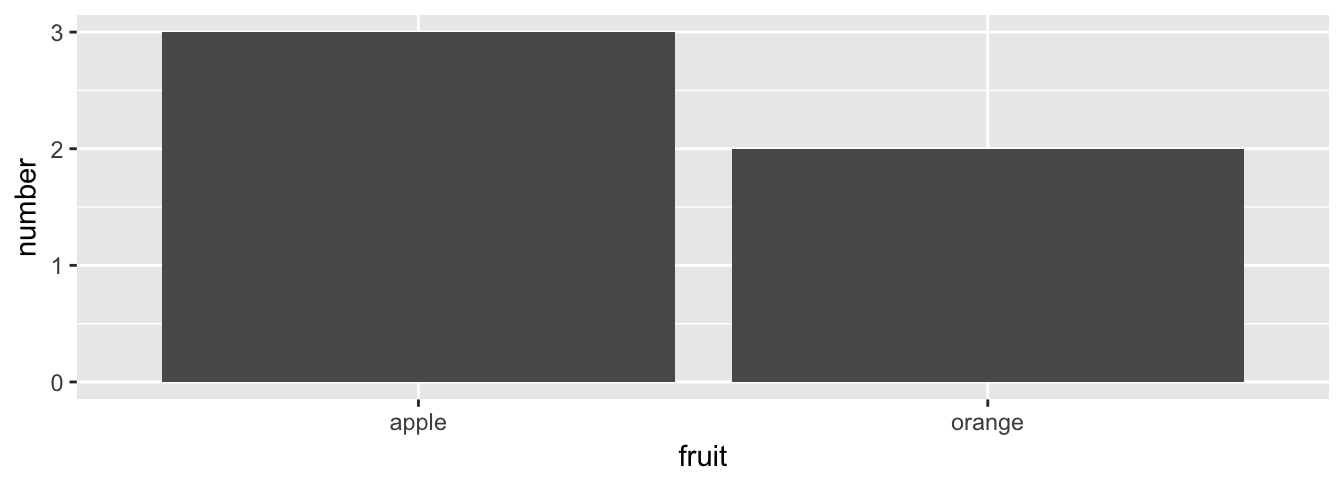Figure 3.15: Barplot when counts are pre-tabulated

Observe that:

• The code that generates Figure 3.14 based on fruits does not have an explicit y aesthetic and uses geom_bar()
• The code that generates Figure 3.15 based on fruits_counted has an explicit y aesthetic (to the variable number) and uses geom_col()

This one aspect of creating barplots using ggplot2 causes the most initial confusion: when the categorical variable you want to plot is not pre-tabulated in your data frame you need to use geom_bar, but if the categorical variable is pre-tabulated and stored in a variable, you need to use geom_col and explicitly map this variable to the y aesthetic.

### 3.8.1 Barplots via geom_bar/geom_col

Consider the distribution of airlines that flew out of New York City in 2013. Here we explore the number of flights from each airline/carrier. This can be plotted by invoking the geom_bar function in ggplot2:

ggplot(data = flights, mapping = aes(x = carrier)) +
geom_bar()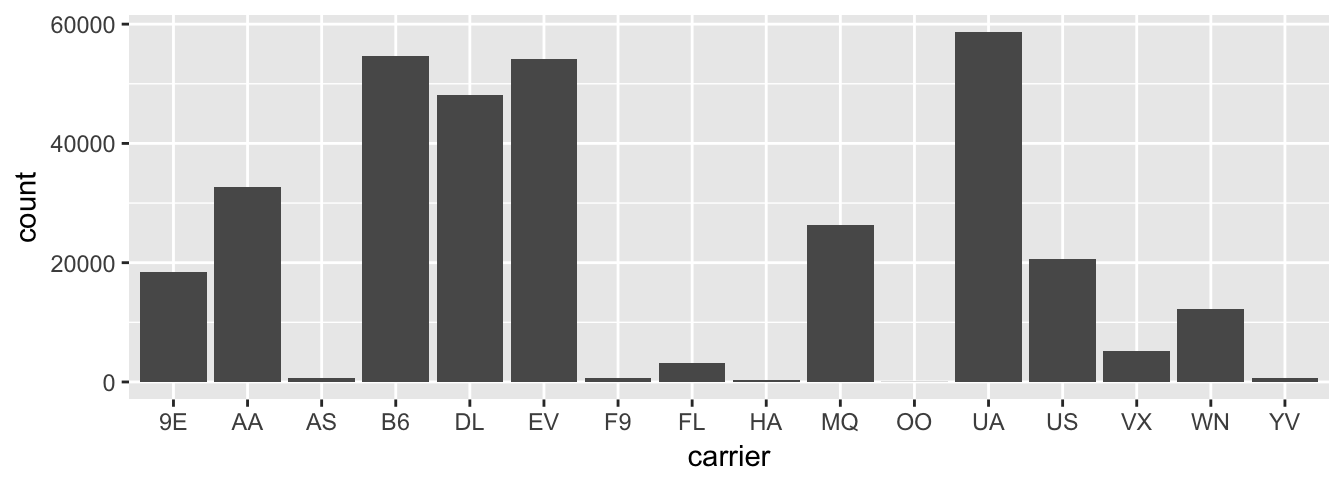Figure 3.16: Number of flights departing NYC in 2013 by airline using geom_bar

To get an understanding of what the names of these airlines are corresponding to these carrier codes, we can look at the airlines data frame in the nycflights13 package. Note the use of the kable function here in the knitr package, which produces a nicely-formatted table of the values in the airlines data frame.

kable(airlines)
carrier name
9E Endeavor Air Inc.
AA American Airlines Inc.
B6 JetBlue Airways
DL Delta Air Lines Inc.
EV ExpressJet Airlines Inc.
F9 Frontier Airlines Inc.
FL AirTran Airways Corporation
HA Hawaiian Airlines Inc.
MQ Envoy Air
OO SkyWest Airlines Inc.
UA United Air Lines Inc.
US US Airways Inc.
VX Virgin America
WN Southwest Airlines Co.
YV Mesa Airlines Inc.

Going back to our barplot, we see that United Air Lines, JetBlue Airways, and ExpressJet Airlines had the most flights depart New York City in 2013. To get the actual number of flights by each airline we can use the group_by(), summarize(), and n() functions in the dplyr package on the carrier variable in flights, which we will introduce formally in Chapter 5.

flights_table <- flights %>%
group_by(carrier) %>%
summarize(number = n())
kable(flights_table)
carrier number
9E 18460
AA 32729
AS 714
B6 54635
DL 48110
EV 54173
F9 685
FL 3260
HA 342
MQ 26397
OO 32
UA 58665
US 20536
VX 5162
WN 12275
YV 601

In this table, the counts of the carriers are pre-tabulated. To create a barchart using the data frame flights_table, we use geom_col and map the y aesthetic to the variable number. Compare this barplot using geom_col in Figure 3.17 with the earlier barplot using geom_bar in Figure 3.16. They are identical.

ggplot(data = flights_table, mapping = aes(x = carrier, y = number)) +
geom_col()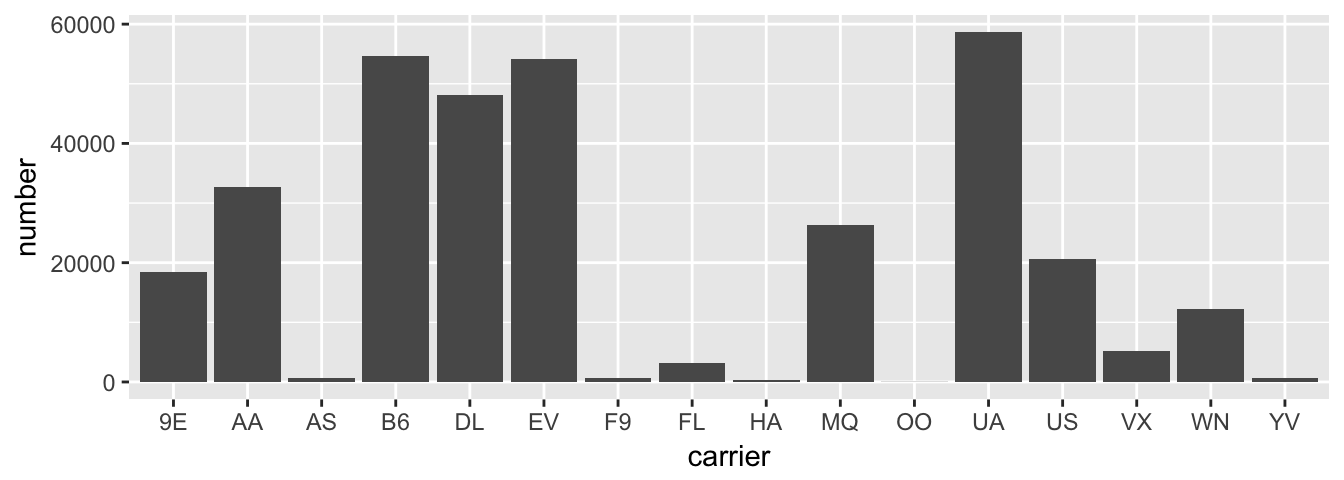Figure 3.17: Number of flights departing NYC in 2013 by airline using geom_col

Learning check

(LC3.26) Why are histograms inappropriate for visualizing categorical variables?

(LC3.27) What is the difference between histograms and barplots?

(LC3.28) How many Envoy Air flights departed NYC in 2013?

(LC3.29) What was the seventh highest airline in terms of departed flights from NYC in 2013? How could we better present the table to get this answer quickly.

### 3.8.2 Must avoid pie charts!

Unfortunately, one of the most common plots seen today for categorical data is the pie chart. While they may see harmless enough, they actually present a problem in that humans are unable to judge angles well. As Naomi Robbins describes in her book “Creating More Effective Graphs” (Robbins 2013), we overestimate angles greater than 90 degrees and we underestimate angles less than 90 degrees. In other words, it is difficult for us to determine relative size of one piece of the pie compared to another.

Let’s examine our previous barplot example on the number of flights departing NYC by airline. This time we will use a pie chart. As you review this chart, try to identify

• how much larger the portion of the pie is for ExpressJet Airlines (EV) compared to US Airways (US),
• what the third largest carrier is in terms of departing flights, and
• how many carriers have fewer flights than United Airlines (UA)?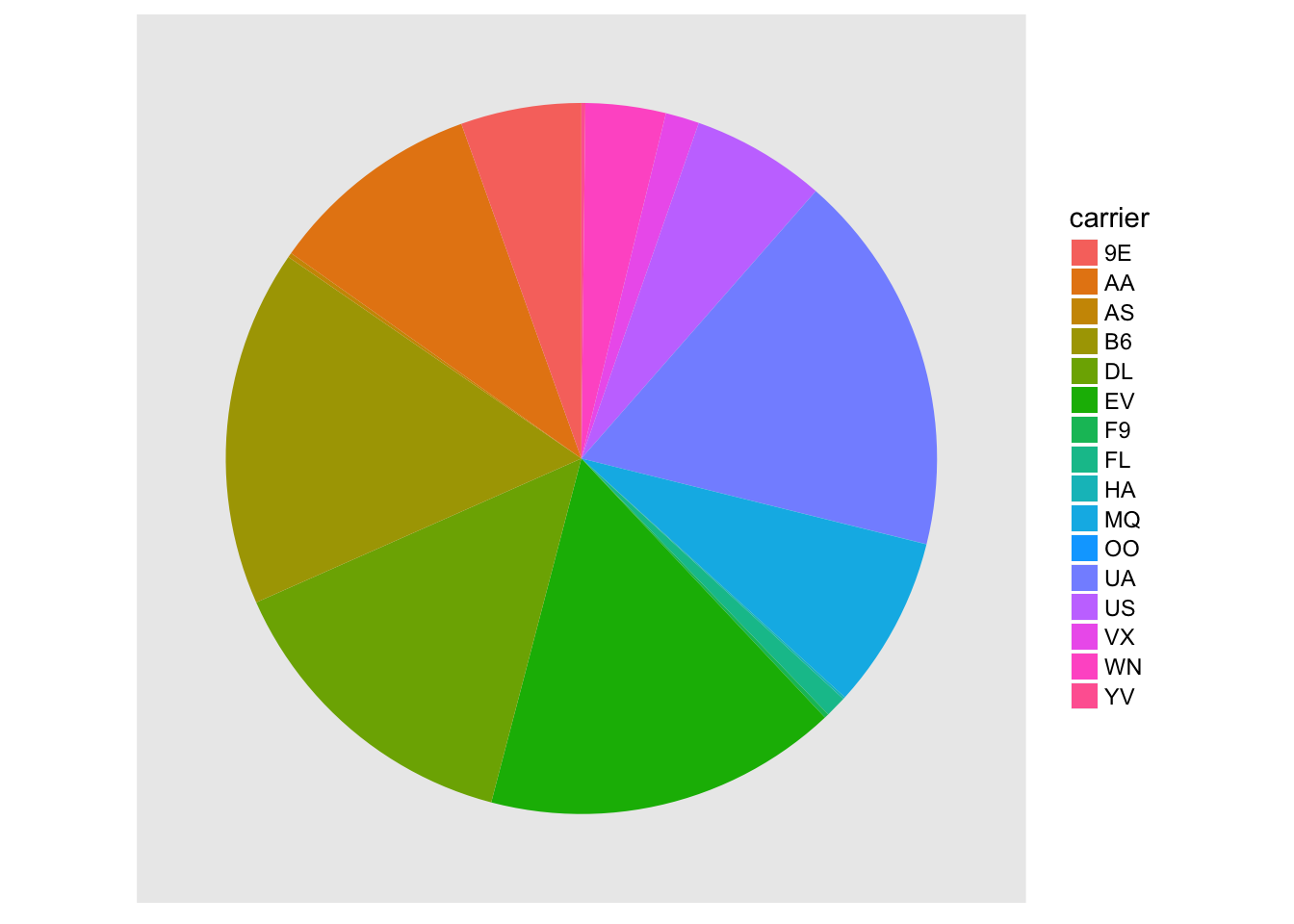Figure 3.18: The dreaded pie chart

While it is quite easy to look back at the barplot to get the answer to these questions, it’s quite difficult to get the answers correct when looking at the pie graph. Barplots can always present the information in a way that is easier for the eye to determine relative position. There may be one exception from Nathan Yau at FlowingData.com but we will leave this for the reader to decide: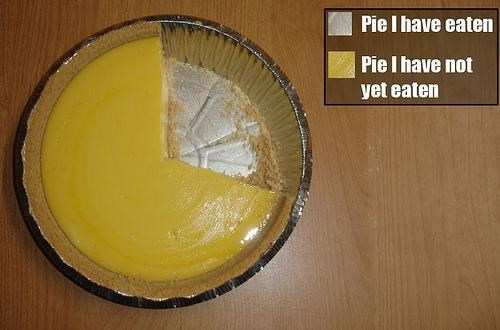Figure 3.19: The only good pie chart

Learning check

(LC3.30) Why should pie charts be avoided and replaced by barplots?

(LC3.31) What is your opinion as to why pie charts continue to be used?

### 3.8.3 Using barplots to compare two categorical variables

Barplots are the go-to way to visualize the frequency of different categories of a categorical variable. They make it easy to order the counts and to compare the frequencies of one group to another. Another use of barplots (unfortunately, sometimes inappropriately and confusingly) is to compare two categorical variables together. Let’s examine the distribution of outgoing flights from NYC by carrier and airport.

We begin by getting the names of the airports in NYC that were included in the flights data-set. Here, we preview the inner_join() function from Chapter 5. This function will join the data frame flights with the data frame airports by matching rows that have the same airport code. However, in flights the airport code is included in the origin variable whereas in airports the airport code is included in the faa variable. We will revisit such examples in Section 5.3 on joining data-sets.

flights_namedports <- flights %>%
inner_join(airports, by = c("origin" = "faa"))

After running View(flights_namedports), we see that name now corresponds to the name of the airport as referenced by the origin variable. We will now plot carrier as the horizontal variable. When we specify geom_bar, it will specify count as being the vertical variable. A new addition here is fill = name. Look over what was produced from the plot to get an idea of what this argument gives.

ggplot(data = flights_namedports, mapping = aes(x = carrier, fill = name)) +
geom_bar()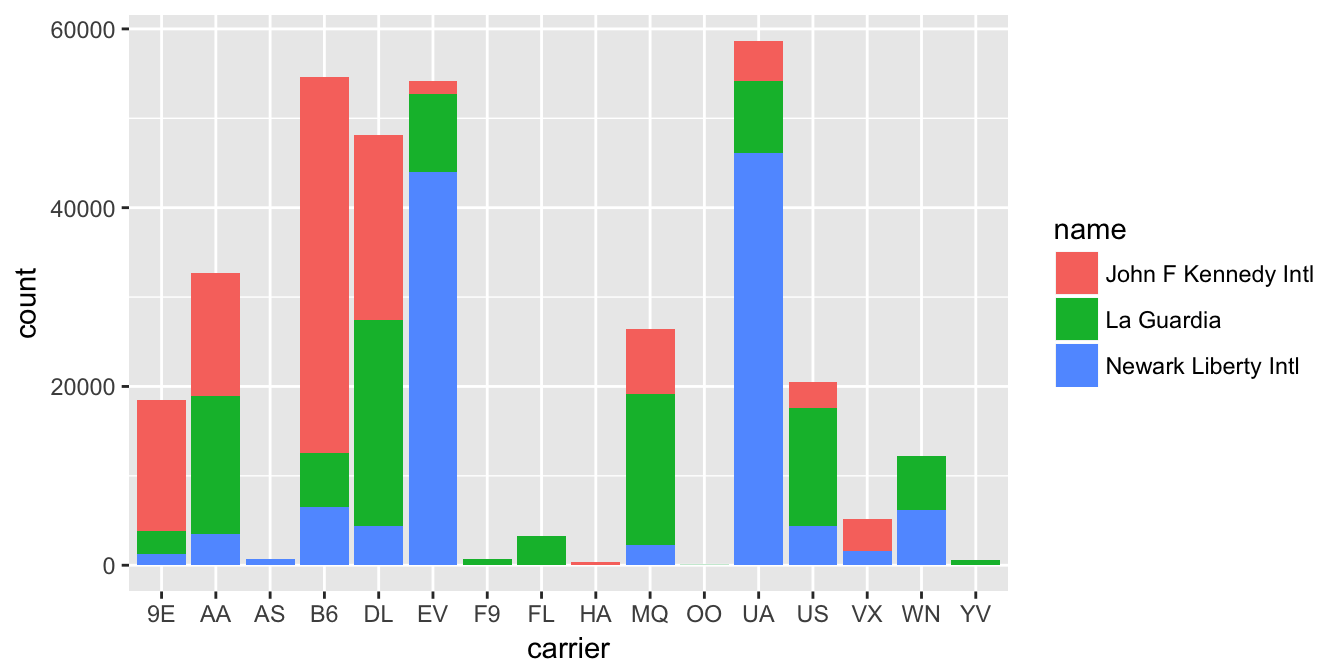Figure 3.20: Stacked barplot comparing the number of flights by carrier and airport

Note that fill is an aesthetic just like x is an aesthetic, and thus must be included within the parentheses of the aes() mapping. The following code, where the fill aesthetic is specified on the outside will yield an error. This is a fairly common error that new ggplot users make:

ggplot(data = flights_namedports, mapping = aes(x = carrier), fill = name) +
geom_bar()

This plot is what is known as a stacked barplot. While simple to make, it often leads to many problems. For example in this plot, it is difficult to compare the heights of the different colors (corresponding to the number of flights from each airport) between the bars (corresponding to the different carriers).

Learning check

(LC3.32) What kinds of questions are not easily answered by looking at the above figure?

(LC3.33) What can you say, if anything, about the relationship between airline and airport in NYC in 2013 in regards to the number of departing flights?

Another variation on the stacked barplot is the side-by-side barplot.

ggplot(data = flights_namedports, mapping = aes(x = carrier, fill = name)) +
geom_bar(position = "dodge")Figure 3.21: Side-by-side barplot comparing the number of flights by carrier and airport

Learning check

(LC3.34) Why might the side-by-side barplot be preferable to a stacked barplot in this case?

(LC3.35) What are the disadvantages of using a side-by-side barplot, in general?

Lastly, an often preferred type of barplot is the faceted barplot. We already saw this concept of faceting and small multiples in Section 3.6. This gives us a nicer way to compare the distributions across both carrier and airport/name.

ggplot(data = flights_namedports, mapping = aes(x = carrier, fill = name)) +
geom_bar() +
facet_grid(name ~ .)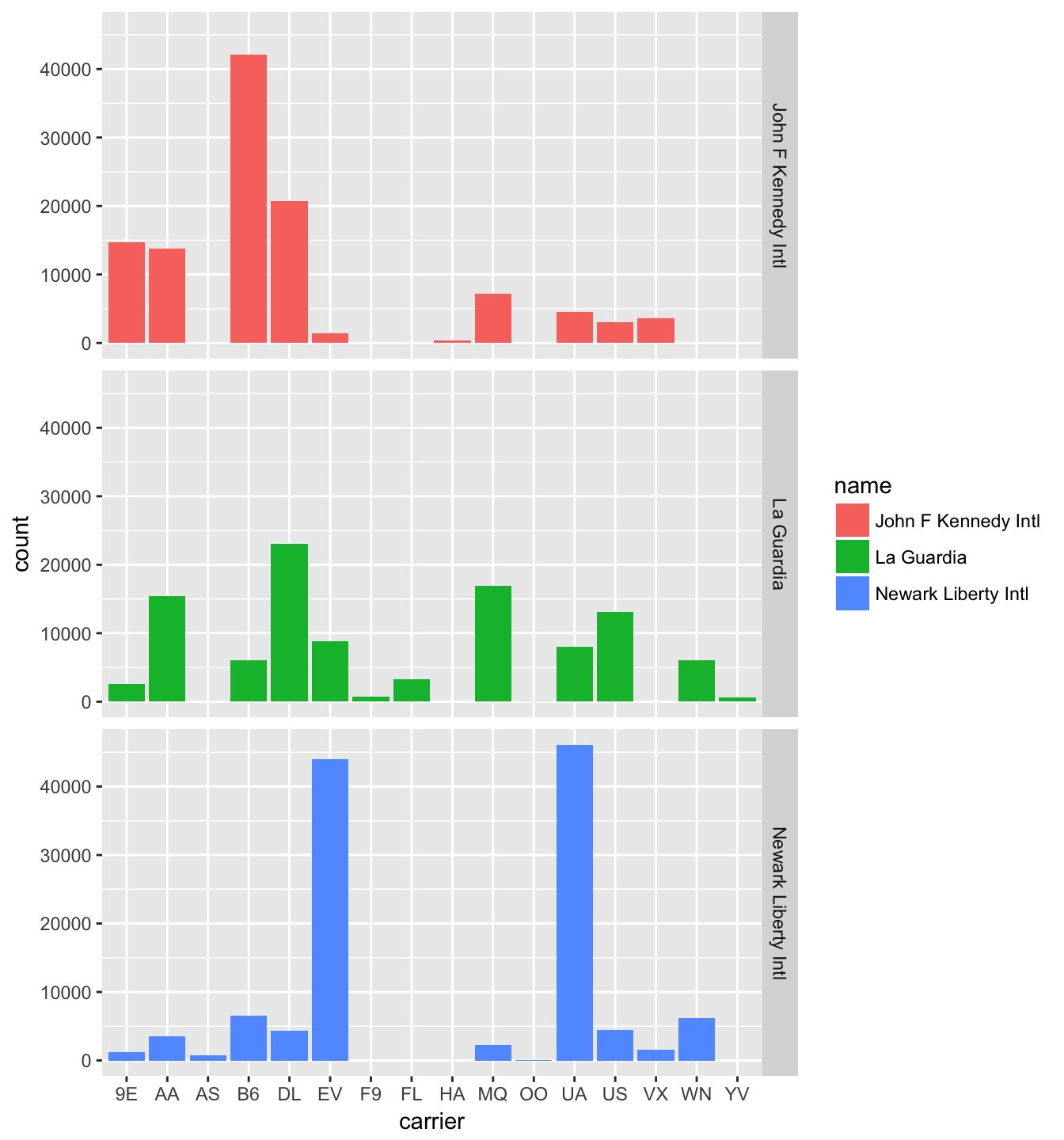Figure 3.22: Faceted barplot comparing the number of flights by carrier and airport

Note how the facet_grid function arguments are written here. We are wanting the names of the airports vertically and the carrier listed horizontally. As you may have guessed, this argument and other formulas of this sort in R are in y ~ x order. We will see more examples of this in Chapter 6.

If you’d like to create small multiples in a vertical direction, you’ll want to use facet_grid() with the name of the variable before the ~ as we did in Figure 3.22. This corresponds to vertical going with y in the formula. If instead you’d like the small multiples to be in the horizontal direction, you’d use facet_grid() with the name of the variable after the ~, corresponding to the x position in the formula. Further, you can use facet_wrap() if you would like the small multiples to wrap into multiple rows as we saw earlier in the faceted histogram example in Figure 3.11. Additionally, you could use facet_grid() with one variable in the y position and another variable in the x position creating a grid of all possible combinations of the two variables.

Learning check

(LC3.36) Why is the faceted barplot preferred to the side-by-side and stacked barplots in this case?

(LC3.37) What information about the different carriers at different airports is more easily seen in the faceted barplot?

### 3.8.4 Summary

Barplots are the preferred way of displaying categorical variables. They are easy-to-understand and make it easy to compare across groups of a categorical variable. When dealing with more than one categorical variable, faceted barplots are frequently preferred over side-by-side or stacked barplots. Stacked barplots are sometimes nice to look at, but it is quite difficult to compare across the levels since the sizes of the bars are all of different sizes. Side-by-side barplots can provide an improvement on this, but the issue about comparing across groups still must be dealt with.

## 3.9 Conclusion

### 3.9.1 Review questions

Review questions have been designed using the fivethirtyeight R package (Ismay and Chunn 2017) with links to the corresponding FiveThirtyEight.com articles in our free DataCamp course Effective Data Storytelling using the tidyverse. The material in this chapter is covered in the chapters of the DataCamp course available below:

### 3.9.2 What’s to come?

In Chapter 4, we’ll introduce the concept of “tidy data” and how it is used as the driving force behind data visualizations and the remainder of the textbook. You’ll see that the concept appears to be simple, but actually can be a little challenging to decipher without careful practice. We’ll also investigate how to import CSV (comma-separated value) files into R using the readr package.

### 3.9.3 Resources

An excellent resource as you begin to create plots using the ggplot2 package is a cheatsheet that RStudio has put together entitled “Data Visualization with ggplot2” available

• by clicking here or
• by clicking the RStudio Menu Bar -> Help -> Cheatsheets -> “Data Visualization with ggplot2

This cheatsheet covers more than what we’ve discussed in this chapter but provides nice visual descriptions of what each function produces.

In addition, we’ve created a mind map to help you remember which types of plots are most appropriate in a given situation by identifying the types of variables involved in the problem.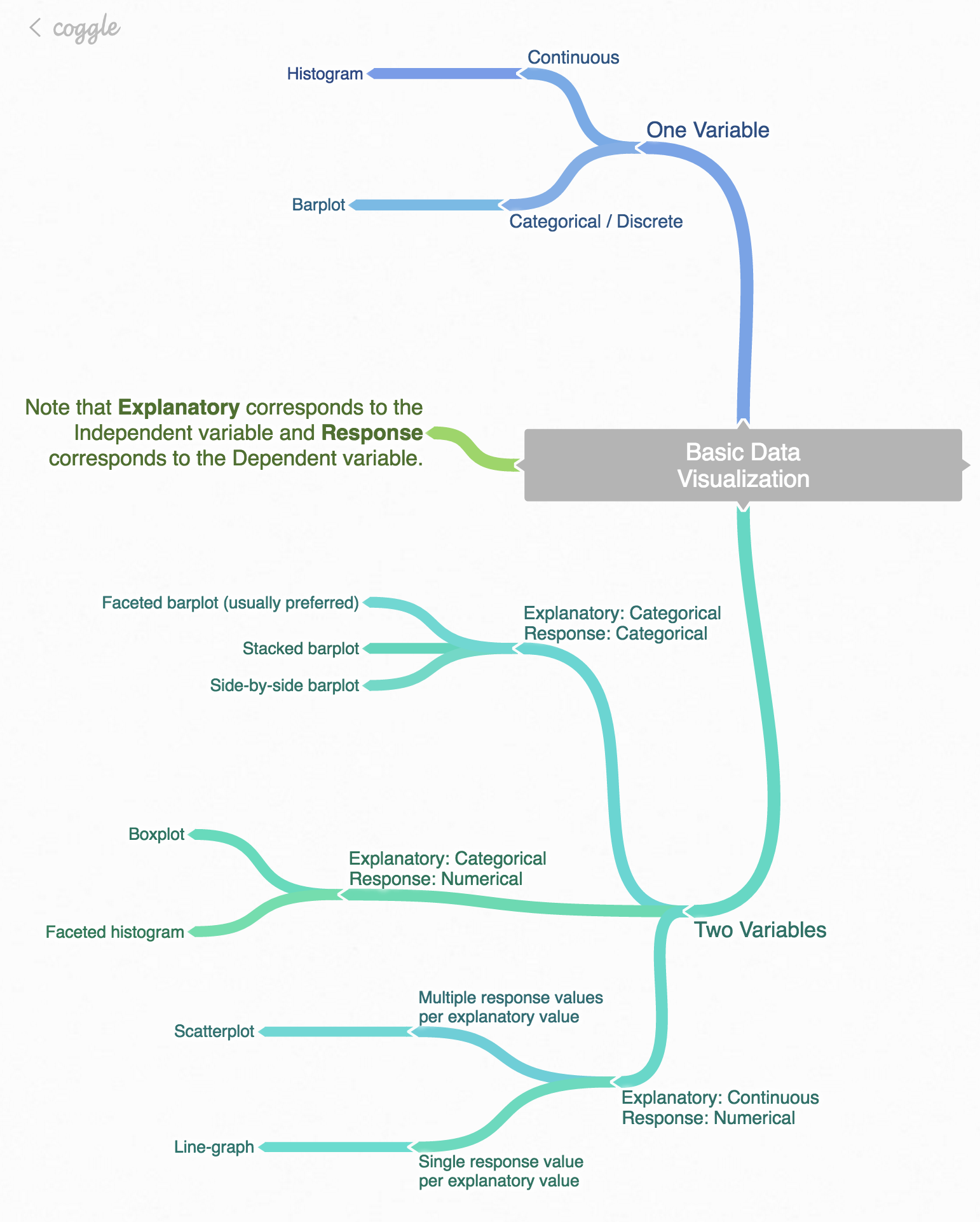Figure 3.23: Mind map for Data Visualization

### 3.9.4 Script of R code

An R script file of all R code used in this chapter is available here.